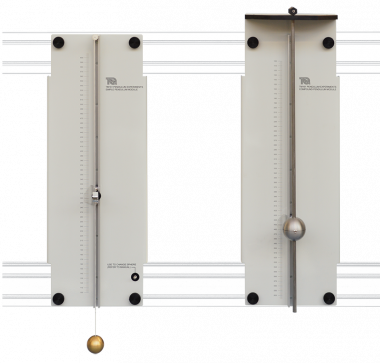TM161 - Experiment

# SIMPLE AND COMPOUND PENDULUMS

PDF datasheet

An experimental apparatus that allows students to study simple harmonic motion and the factors that affect the period of oscillation of pendulums.

OR CALL US TO DISCUSS+44 1159 722 611

SIMPLE AND COMPOUND PENDULUMS

This product is part of a range that explores free vibrations in simple ‘one degree of freedom’ systems.

It introduces students to key scientific terms such as:

• Simple harmonic motion (SHM) and period of oscillation
• Mass moment of inertia
• Routh’s rule

This product fits to the sturdy Test Frame (TM160) for study or demonstration.

This product includes a simple pendulum and a compound pendulum. Both pendulums swing through small angles, showing the principles and use of simple harmonic motion using the small angle approximation.

The simple pendulum uses a cord which may be considered as ‘light’ so you can ignore its mass, leaving only the sphere and cord length as the important variables.

The compound or ‘physical’ pendulum uses a ‘heavy’ solid rod which has reasonable mass, so you must allow for it in theoretical equations.
Each pendulum has a back panel that fixes separately to the Test Frame. The back panel of each pendulum has an accurate scale and indicator, referenced to pendulum pivot or centre of mass points. This improves measurement accuracy - essential for good results.

The simple pendulum has a choice of two spheres suspended by a cord. An adjustable indicator also acts as the pendulum pivot point - allowing you to adjust the cord length in seconds. This can also provide a quick visual demonstration of the effect of cord length on period. Each sphere has a different mass for comparison and an internal spring retainer so you can easily swap them between experiments.

The compound pendulum has a solid rod that pivots on a hardened steel knife edge on the upper surface of a steel platform. This pendulum includes an additional spherical mass that fixes at any position along the rod. It also includes an extra pivot and disc-shaped mass so you can convert it for use as a Kater’s pendulum.

The Kater’s pendulum works as a reversible and adjustable compound pendulum. Students adjust the relative positions of its parts until they have equal time periods in both its standard and reversed arrangements. It then works as an accurate gravimeter.

Students test different pendulums to see how different factors, such as mass or pendulum length affect simple harmonic motion and the period of oscillation. The theory shows how to predict the period of oscillation of a given pendulum for comparison with actual results. It also shows how pendulums can produce an approximate value of the acceleration due to gravity.

VIDEO
• Cord length and period of a simple pendulum.
• Mass and period of a simple pendulum.
• Using a simple pendulum to find the acceleration due to gravity.
• Centre of gravity and period of a compound pendulum.
• How an adjustable mass affects the period of a compound pendulum.
• Using a Kater’s pendulum to find the acceleration due to gravity.
SIMPLE AND COMPOUND PENDULUMS
SUPPORTING PRODUCTS
TM160 - Base Unit

A sturdy frame for use with TecQuipment’s Free Vibrations Experiments.

ES7 - Experiment

Demonstrates simple harmonic motion (oscillation) in springs and pendulums, and its usefulness.